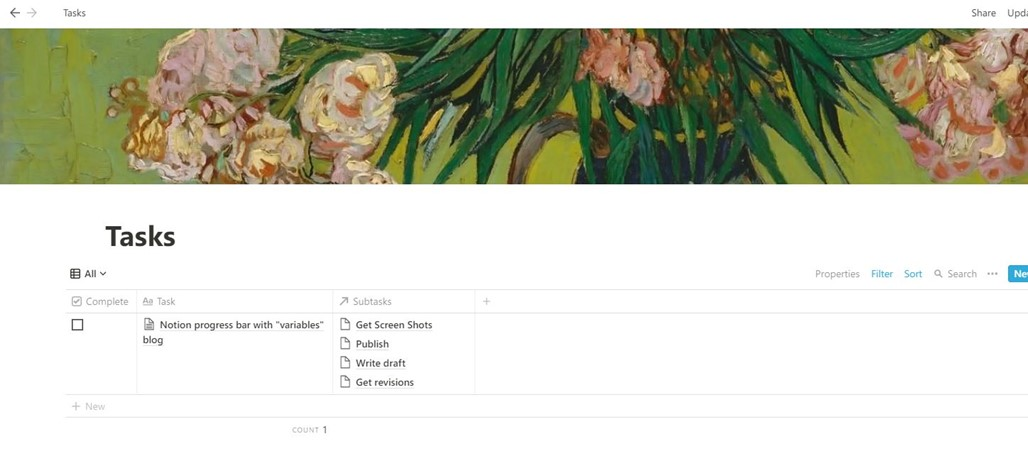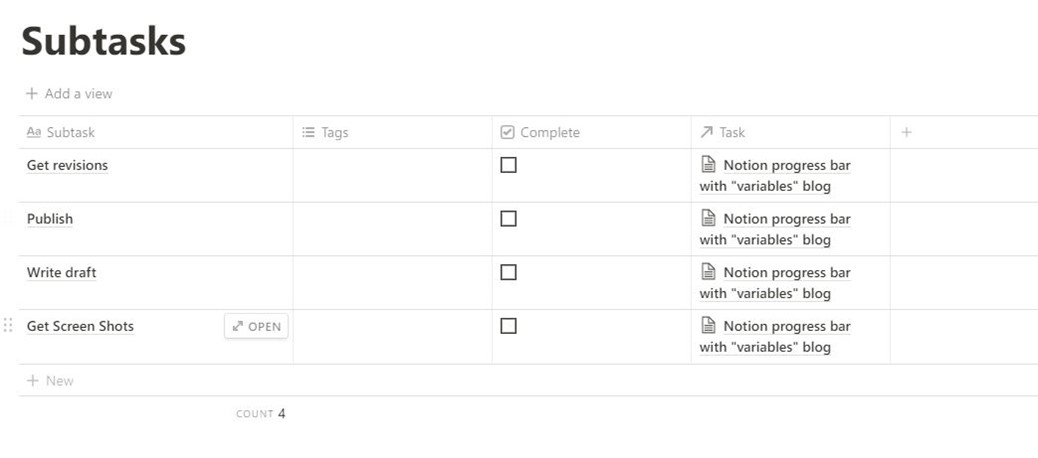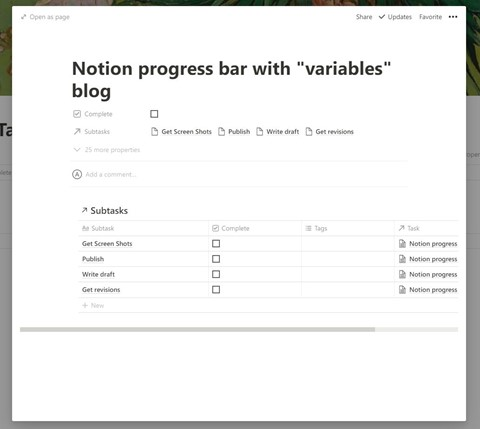# Creating a Notion Progress Bar with Variable Formulas

## Don’t Copy Formulas: Make Them Yourself With this Power-user Technique!

The beauty of Notion.so is that it offers so much flexibility in how you can manage and view your data. Much of this flexibility comes from the column Formula tool. While the possibilities of column data formatting and calculations with a Notion Formula are endless, the provided formula editor is very cumbersome and limited, with no syntax correction. When a formula grows, it becomes easy to miss a comma or a parenthesis and get stuck trying to find your error. A power-user solution is to nest formulas within formulas by turning them into variables.

### Disclaimer

This blog demonstrates making a Notion progress bar built with readable, manageable, and reusable formulas. If you want a formula for a one-off progress bar, then this is not the blog for you. However, if you want to learn how to be a Notion Formulas power user and understand for yourself how to make excellent formulas fast, then follow along.

Note: if you just want that “copy and paste” progress bar formula, here it is:

``format(slice("▓▓▓▓▓▓▓▓▓▓", 0, floor(prop("_progress") * 10)) + format(slice("░░░░░░░░░░", 0, ceil(10 - prop("_progress") * 10))))``

### Notion Formula Pro Tip

Notion Formulas are written in JavaScript so you can use an online editor like jsfiddle.net to edit your formulas, take advantage of syntax highlighting and keep track of parenthesis!

### Building a Notion Progress Bar

#### Database Setup

To have a progress bar, we first need a one-to-many relationship. In this example, we use a `task` that can have zero or more `subtasks`. We set up this relationship by creating two databases—one for `tasks` and one for `subtasks` After that, we create a relation property connecting the `tasks` database to the `subtasks` database.The tasks database with columns for “Complete” (a checkbox property), “Task” (The task’s name), and the associated “Subtasks.”Similarly, the subtask database has columns for “Complete” (a checkbox property), “Subtask” (The subtask’s name), and the associated “Task.”

Now we can create a task and add a view to show the subtasks associated with it. If stuck, you can read about the fundamentals of connecting databases with a relation property.Now, let’s start making the Notion progress bar that shows us how many subtasks we have completed!

### Ternary Operator Tip Box

This blog’s formulas heavily rely on the ternary operator, denoted by a “?” question mark character.

It works like this:

``````value = (if this statement in the parenthesis is true) ?
(set "value" to this) :
(else set "value" to this thing on the right side of the colon)``````
``````//Example:
color = true ? red : blue
//color is set to red.
//Example:
color = false? red : blue
//color is set to blue.``````

Once you see the ternary operator `?` in a formula’s code, you can refer to the pseudocode example for a reminder.

### Breaking Down the Formula

We want to make the final progress bar formula as simple as possible by breaking down the required calculations into chunks. In other words, we want to build it step by step. We will break it down into 7 properties for demonstrating the idea of variable properties.

#### Step 1: Get the Completed Subtasks to Total Subtasks Ratio

We first need to get the ratio of completed subtasks to total subtasks for a Notion progress bar.

We start by creating a variable property to store the total number of subtasks a task has; do this with a rollup that counts the number of subtasks.

#### 1. `_numSubTasks`:

Also, we can name our variable property starting with a “_” underscore to denote that it is a variable property and is not intended to be viewed in the table. This also helps with organization and clutter as it puts it at the bottom of the properties list when managing our table’s properties.

Next, we can create a variable property called `_numSubtasksComplete` that stores the number of completed subtasks. We will do this with a rollup that counts the number of checked-off subtasks.

#### 2. ` _numSubtasksComplete`:

Some tasks have subtasks, while others may not have subtasks (a progress bar doesn’t make much sense for a one-off task). We want the Notion progress bar to look differently for both situations, so we create a simple variable to store a true or false value of whether the task has subtasks.

#### 3. `_hasSubTask`:

``prop("_numSubTasks") > 0``

Now we can create a variable property to store the ratio of completed tasks.

#### 4. `_progress`:

``````prop("_hasSubTask") ?
toNumber(prop("Complete"))``````

`toNumber(prop("Complete"))` turns the “Complete” checkbox property (see the “Database Setup” section), which is either a `true` or a `false`, into a 1 or a 0, respectively, so that the result is a number in either case of the ternary statement.

#### Step 2: Create “Views”

Now, create a property that you can call a “view.” A view in software design is a chunk of code with the specific responsibility of making content or data visible to a user in a friendly way. Our view renders a formatted ratio of our completed subtasks to total subtasks (using the variable properties we created in step 1) and is only intended for viewing (and not to be used in other formulas as a variable for calculations). In calling it a view, we can remember its intended function: viewing.

#### 5. `_view_RatioTasksCompleted`:

``````prop("_hasSubTask") ? concat(format(prop("_numSubTasksComplete")), "/",
format(prop("_numSubTasks"))) : (prop("Complete") ? "1/1" : "0/1")``````

The above formula checks first if a task has subtasks. If so, it will output the ratio of tasks completed. If not, it checks whether the task has been marked “Complete” and creates a “1/1” or “0/1” output.

Note: consider how much longer the formula associated with our `_view_RatioTasksCompleted` property would have been if we had not used our nice variables that have descriptive names.

Now we can create our Notion progress bar “view.” We can reuse this simple progress bar view property anywhere we want, as all it relies on is a single ratio input (in our case, our _progress variable).

#### 6. `_view_ProgressBar`:

``````format(slice("▓▓▓▓▓▓▓▓▓▓", 0, floor(prop("_progress") * 10)) +
format(slice("░░░░░░░░░░", 0, ceil(10 - prop("_progress") * 10))))``````

Note: the Formula outputs text characters that look like a progress bar. You can change what character to use for filled and unfilled portions of them by editing the slice(“*****“… part of the formula.

#### Step 3: Put It All Together

Finally, we can create an easy-to-read progress bar view property composed of `_view_ProgressBar` and the `_view_RatioTasksCompleted`:

``````((prop("_view_ProgressBar") + " " +

Depending on whether `_hasSubTask` is true or false, we can show a progress bar or only the simple ratio in our final `Progress` view property:

#### 7. `Progress`:

``````prop("_hasSubTask") ?
(prop("_view_ProgressBar") + " " + prop("_view_RatioTasksCompleted")) :

It outputs our progress bar `_view_ProgressBar` view property with a space `" "` followed by the `_view_RatioTasksCompleted`:

If the task has no subtasks, then the view outputs only the `_view_RatioTasksCompleted` :

### You Did It!

You can use this technique of variable properties to scale your formulas to immense complexity, and you can test at each step to make sure your code is working as intended. The downside is that you end up with many properties to hide (remember to use a naming convention like starting variable property names with an underscore, e.g., “_variableName,” to keep track of them).

If you have questions, feel free to comment below!

### Want More Notion Tips and Tricks?

Read more about “variables” and Notion fundamentals in this cheat sheet article.

If you are also a Microsoft Teams user and are interested in a robust project documentation solution, check out IntelliWiki!

Happy Notion’ing!